# Double Diffusive Natural Convection in Binary Mixture Under The Effect of External Magnetic Field: Steady and Oscillatory State

Double Diffusive Natural Convection in Binary Mixture Under The Effect of External Magnetic Field: Steady and Oscillatory State

Badia Ghernaout Said BouabdallahMohamed Teggar Hamza Benniche

LME, Laboratory of Mechanics, University of Laghouat, BP.37G, Laghouat, 03000, Algeria

Corresponding Author Email:
fibonsaid@gmail.com
Page:
11-18
|
DOI:
http://dx.doi.org/10.18280/ijht.330402
|
Accepted:
|
Published:
31 December 2015
| Citation

OPEN ACCESS

Abstract:

In this paper, double diffusive natural convection in binary mixture under the effect of external magnetic field for the steady and the oscillatory state is numerically investigated. For this end, a square cavity filled with a binary mixture has been considered; the cavity is exposed to opposing solute and thermal gradients. An external uniform magnetic field is applied separately in two directions (i.e. horizontal and vertical). The following flow parameters were considered: Prandtl number Pr = 0.71, Schmidt number Sc = 3.5 and buoyancy ratio N = 0.75, 1.0, and 1.25. The finite volume method with SIMPLER Algorithm was used to solve numerically the mathematical model. The model was validated and showed good agreement with experimental results of the literature. The obtained results showed a strong dependence of the structure of thermal and solutal effect to the buoyancy ratio. The oscillatory double diffusive flow occurs for a periodic time where the phenomenon change around in each period time. A critical thermal Rayleigh number RaTCr and corresponding dominated frequency for the onset of oscillatory double diffusive convection were determined for each N and for different values of Hartman number (0, 25, 50, and 100).

Keywords:

Buoyancy ratio, Critical value, Double diffusive convection, Magnetic field, Oscillatory flows.

1. Introduction

The electromagnetic control of the thermal and solutal convection in the liquid phase was widely used in the processes of crystal growth of semiconductors. It consists, by the application of a magnetic field, of stabilizing the flow and in cancelling the instabilities. The electromagnetic amortization of the convective flows was studied numerically and experimentally for flows in rectangular 2D cavities (Hurle et al.  and Hof et al. ) and in 3D configurations (Okada and Ozoe ; and Di Pizza and Ciofalo ).

The natural double diffusive convection in the presence of the magnetic field, crystal growth and the flows without phase change (by experimental and numerical ways), the studies have identified a variety of cases involving the application of magnetic fields in: the stability of the flow, the removal of flow, the stability of the interface and the growth rate. For example, the effect of magnetic fields on the flow structure, the interface shape of growth, and the growth rate in the techniques of crystal growth from a solution or a melt (Dost et al. ; Galindo et al. ; Liu et al. ; Seibani et al. ; Kumer et al. ; Yieldiz et al. ; and Armour et Dost ).

Gelfgat and Yoseph  studied the effect of an external magnetic field on oscillatory instability of convective flow in a rectangular cavity with different magnitudes and orientations. Stability diagrams for the dependence of the critical Grashof number on the Hartmann number were obtained by the authors. They showed that a vertical magnetic field provides a stronger stabilization effect, and also that the multiplicity of steady states is suppressed by the electromagnetic effect. During solidification of a pure metal Bessaïh and Bouabdallah  investigated numerically the two-dimensional oscillatory natural convection in a rectangular cavity subjected to an external and arbitrarily oriented magnetic field. The authors showed that the effect of a magnetic field with different orientations provides a notable change on the flow and thermal structures. Stability diagrams were obtained and showed a strong dependence of the critical Grashof number and the dimensionless critical frequency for various values of the Hartmann number. After, Bouabdallah and Bessaih  examined the effect of magnetic field on the flow and the three-dimensional heat transfer during phase change of a liquid metal. They have shown that the magnetic field causes a significant control of the shape of the solid/liquid interface, and for high values of the Hartmann number (Ha = 100), the convection is suppressed and the interface is regular. They have also showed that the magnetic field causes the bidimensionalisation of the heat transfer along isothermal walls. Recently, Ghernaout et al.  carried out the effect of the buoyancy ratio on oscillatory double-diffusive convection in binary mixture. They show that the critical thermal Rayleigh number decrease when the solute effect is dominated, but this value increase when the thermal effect has dominated and the low value of critical thermal Rayleigh number is obtained for the case of equal opposing thermal and solute force.

In the present work, the effect of an external magnetic field on the steady and oscillatory double diffusive natural convection in binary mixture in a square enclosure is studied numerically. We applied the external magnetic field along the horizontal and vertical directions, separately, and this for different Hartmann number values (0, 25, 50, 75 and 100). The walls of the enclosure are considered electrically insulated. This paper is organized as follows: Section 2 presents the problem description and mathematical formulation. Section 3 discusses the numerical method and techniques, which have been used for the computation, the grid independence study, and the comparison between our predictions and the results found in the literature. Section 4 presents the results and discussion: onset of the oscillatory double-diffusive convection flow and mass flow, and the effect of the buoyancy ratio (N: 0.75, 1.0, and 1.25) on critical thermal Rayleigh number are presented and discussed.

2. Problem Description and Mathematical Formulation

The geometry under consideration is a square cavity filled with a binary fluid (Figure 1). Different temperatures and concentrations are specified between the left ($T_{1}$,$C_{1}$) and right vertical walls ($T_{2}$,$C_{2}$), where $C_{1} \phi C_{2}$ and $T_{1} \phi T_{2}$, and zero heat and mass fluxes are imposed on the remaining walls with no slip boundary conditions for all velocity components. The flow is assumed to be laminar and the binary fluid is assumed to be Newtonian and incompressible. In addition, the Soret and Dufour effects are assumed to be negligible and the fluid density to be constant, except in the buoyancy term of the Navier–Stokes equations, where it varies linearly with the local temperature and solute mass fraction as: $\rho=\rho_{0}\left[1-\beta_{T}\left(T-T_{0}\right)-\beta_{C}\left(C-C_{0}\right)\right]$.

Where $\beta_{T}=-\frac{1}{\rho_{0}}\left[\frac{\partial \rho}{\partial T}\right]_{C}$ and $\beta_{C}=-\frac{1}{\rho_{0}}\left[\frac{\partial \rho}{\partial C}\right]_{T}$ . With $\beta_{T} \phi 0$ and $\beta_{C} \pi 0$ . The thermo-physical properties of the fluid are taken as constant and they are estimated at a reference temperature $T_{0}$ and reference solute mass fraction $C_{0}$ , which are set to be equal to $T_{2}$ and $C_{2}$ , respectively.

## 1.png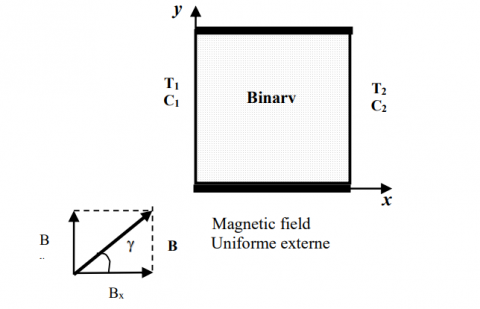Figure 1. Schematic of the physical problem

Using the following dimensionless variables: $X=x / L$ , $Y=y / L$ , $\tau=t /\left(L^{2} / v\right)$ , $V=v /(v / L)$ , $U=u /(v / L)$ , $P=p L^{2} / \rho v^{2}$ , $\theta=\left(T-T_{2}\right) /\left(T_{1}-T_{2}\right)$ , $\Phi=\left(C-C_{2}\right) /\left(C_{1}-C_{2}\right)$ , where $L$ is the length of square cavity,$v$ is the kinematics viscosity of the fluid and $V$ is the velocity vector, the equations governing the conservation of mass, momentum, energy and species concentration in dimensionless form can be written as:

$\frac{\partial U}{\partial X}+\frac{\partial V}{\partial Y}=0$    (1)

$\frac{\partial U}{\partial \tau}+\frac{\partial(U U)}{\partial X}+\frac{\partial(U V)}{\partial Y}=-\frac{\partial P}{\partial X}+\nabla^{2} U+F_{E M X}$     (2)

$\frac{\partial V}{\partial \tau}+\frac{\partial(U V)}{\partial X}+\frac{\partial(V V)}{\partial Y}=-\frac{\partial P}{\partial Y}+\nabla^{2} V+\frac{R a_{T}}{\operatorname{Pr}}(\theta-N \Phi)+F_{E M Y}$     (3)

$\frac{\partial \theta}{\partial \tau}+V \cdot \nabla \theta=\frac{1}{\operatorname{Pr}} \nabla^{2} \theta$     (4)

$\frac{\partial \Phi}{\partial \tau}+V . \nabla \Phi=\frac{1}{L e \operatorname{Pr}} \nabla^{2} \Phi$   (5)

Where $F_{E M X}$ and F_{E M Y} represent the components of Lorentz force equation: F=J \times B (Gelfgat and Yoeseph, ; Bessaih and Bouabdallah, ), where J, B are the electric current and magnetic field vectors, respectively in X and Y directions respectively, which are obtained using as:

$F_{E M X}=H a^{2}\left[V \cos (\gamma) \sin (\gamma)-U \sin ^{2}(\gamma)\right]$    (6.a)

$F_{E M Y}=H a^{2}\left[U \cos (\gamma) \sin (\gamma)-V \cos ^{2}(\gamma)\right]$       (6.b)

$H a=B_{0} L \sqrt{\sigma / \rho v}$ , the Hartmann number $R a_{T}=\left(g \beta_{T} \Delta T L^{3}\right) / v \alpha$ , the thermal Rayleigh number, $N=R a_{S} / R a_{T} L e$ is the ratio of the buoyancy forces, $R a_{S}=\left(g \beta_{C} \Delta C L^{3}\right) / v D$ , the solutal Rayleigh number, $\mathrm{Pr}=v / \alpha$ the Prandtl number, $L e=\alpha / D$ , the Lewis number, $\alpha$ and $D$ are the thermal and molecular diffusivities respectively. The initial and boundary conditions are given as:

For $\tau=0 ; U=V=\Theta=\Phi=0$

For $\tau \phi 0$ ;   (7a)

At $X=0$ and $X=1,0 \leq Y \leq 1: U=V=0$   (7b)

At $Y=0$ and $Y=1,0 \leq X \leq 1: U=V=0, \frac{\partial \theta}{\partial Y}=\frac{\partial \Phi}{\partial Y}=0$   (7c)

At $X=0,0 \leq Y \leq 1: \theta=\Phi=0$  (7d)

At $X=1, \quad 0 \leq Y \leq 1: \theta=\Phi=1$   (7e)

The average heat and mass fluxes at the left wall are given in the dimensionless terms by Nusselt and Sherwood numbers as:

$\overline{N u}=\int_{0}^{1}\left(\frac{-\partial \theta}{\partial X}\right) d Y$   (8a)

$\overline{S h}=\int_{0}^{1}\left(\frac{-\partial \Phi}{\partial X}\right) d Y$     (8b)

3. Numerical Solution Process

3.1 Numerical method

The set of equations (Eq.1-5) associated to the boundary conditions (Eqs.7a-e), were discretized by a finite volume method Patankar . The SIMPLER algorithm was applied to solve the velocity-pressure coupling. The convective and diffusive fluxes were approximated by a second-order accurate central differencing scheme. The discretized algebraic equations were solved by the line-by-line Tri-Diagonal Matrix Algorithm (TDMA).

## 2a.png(a)

## 2b.png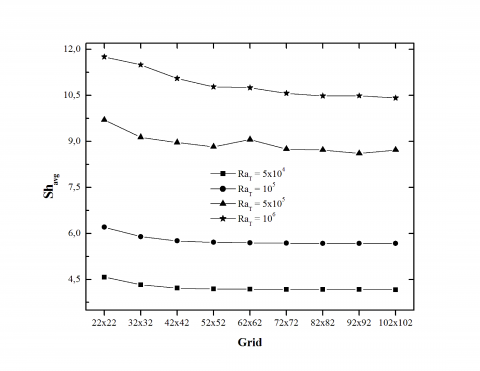(b)

Figure 2. Effect of grid size on the heat rate, Nuavg (a) and mass transfer rate, Shavg (b), for N = 1.25, Pr = 10 and Le = 10

A fully implicit time marching was realized with a fixed dimensionless time step (Dt = 5×10-6). At this stage, the steady-state solution was reached. Calculations were carried out on a Work Station (CPU Core 2 Quad Q9625, 3.00 GHZ-12 Mo. L2); the average computing time for a typical case was approximately 7 hours.

To test and assess the grid independence study, nine irregular grids were tested: 22×22, 32×32, 42×42, 52×52, 62×62, 72×72, 82×82, 92×92 and 102×102 for $\varepsilon$=1.07 ($\varepsilon$ represents the coefficient of geometric progression). In Figure 2, the influence of the number of grids on the average numbers of Nusselt and Sherwood is illustrated for: N = 1.25 , Pr = 10, Le = 10 and for various Rayleigh number values RaT = 5×104, 105, 5×105 and 106. It seems that the rates of the heat and mass transfer become insensitive to the grid 72x72. Along the present work, we will adopt, for reasons of compromise precision / time computing, the grid 82×82.

3.2 Validation of the computer code

In order to verify the accuracy of the present numerical study, the computer code developed in this study was validated with the numerical results. A good agreement was obtained with the results of Beghein et al.  and Sezai and Mohamed . That, we have reported in the work of Ghernaout et al. . In this work, a validation was carried out by the comparison between our results and the experimental data of Han and Kuehn  and Han and Kuehn . The case of natural convection with double diffusion where the forces of volumes are opposing and with the following parameters: A = H / L = 4, Pr = 8, Sc = 2000, GrT = 4×105and GrS = 3×106 are considered. Figures 3a-b presents the variation of the dimensionless temperature and concentration in the half-length of the enclosure of our results and those of Han and Kuehn . These figures show a good agreement between the numerical and experimental results with a light difference on the level of the horizontal walls (adiabatic walls), which can be explained by the non-idealist of the adiabatic condition implied on the horizontal walls.

## 3a.png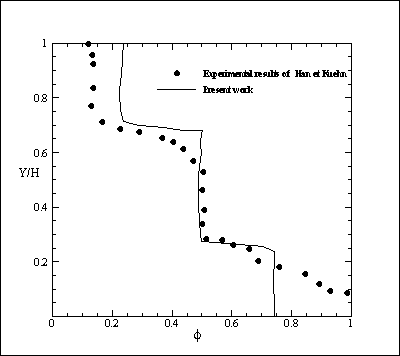(a)

## 3b.png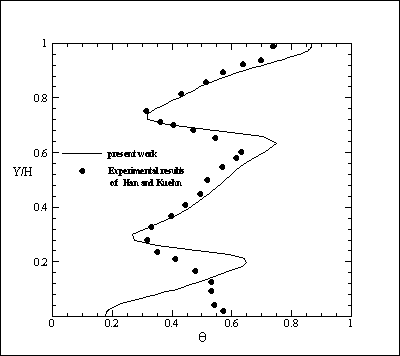(b)

Figure 3. Comparison between our results and the experimental results of Han and Kuehn (1991), profiles of the temperature at X = L/2 (a), and the concentration at X = L/2 (b), for: A = H / L = 4, Pr = 8, Sc = 2000, GrT = 4×105 and GrS = 3×106

4. Results and Discussions

In this section, we present the numerical results of the effect of magnetic field on the double diffusive convection flow in the enclosure. The following flow parameters were considered: Prandtl number Pr = 0.71, Schmidt number Sc = 3.5 and buoyancy ratio N = 1.75 , 1.0, and 1.25. We varied the Hartmann number from 0 to 100 (Ha = 0, corresponds to the case without magnetic field). All variables U, V, $\theta$ and $\phi$ are given in dimensionless form.

4.1 Steady flow in the presence of the magnetic field

With the aim of contributing to the understanding of the effect of amortization of a “static and uniform” magnetic field applied to the double diffusion flow, we carried out numerical tests in which an external magnetic field is applied. Initially, the magnetic field is applied in the horizontal and vertical direction separately, for Prandtl number Pr = 0.71, Schmidt number Sc = 3.5 (the same parameter as the work of Zhou and Zebib, ; N = 0.75 and RaT = 7.5 × 104 $\pi$ RaTCr (laminar steady)). The variation of the Nusselt number (Nu) and Sherwood number (Sh) is illustrated in Figure 4 and this for different values of the Hartmann number (Ha = 0, 5, 10, 20, 30, 50 and 100). The magnetic field is applied in two directions horizontal and vertical, separately. It is found that, more than the Hartmann number increases, the rate of heat transfer and the mass is reduced; such as the Nusselt number decreases at 3.7 (Ha = 0) to a value close to unity (Ha = 100), while the thermal convection is completely eliminated (pure conduction), and this for the two orientations of the magnetic field Bx and By. Moreover, the rate of mass transfer is reduced from Sh = 6.569 (Ha = 0) to Sh = 1.407 (Ha = 100, By). So, by comparing the magnetic stabilization produced by the two types of magnetic field, we can see that the magnetic field By stabilizes better the rate of mass transfer compared to Bx. However, the magnetic field is used to stabilize the double-diffusive convection flow, and that the magnetic field applied in vertical direction has a strong magnetic stabilization.

## 4.png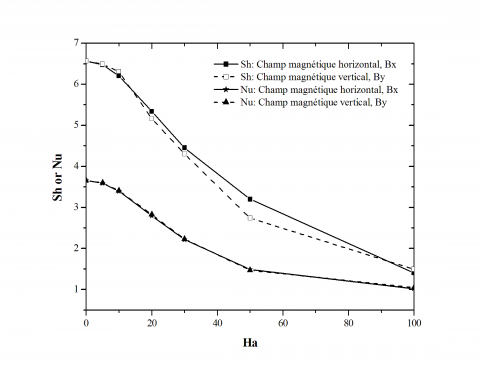Figure 4. Variation of the Nusselt number (Nu) and Sherwood number (Sh), for different values of the Hartmann number for N = 0.75 and RaT = 7.5 × 104 $\pi$ RaTCr (steady state)

4.2 Effect of magnetic field on the oscillatory flow

According to the methodology of the study of an oscillatory flow (unsteady) exposed to an external uniform magnetic field (Gelfgat and Yoeseph, ; Bessaïh and Bouabdallah, ; Bouabdallah and Bessaih, ; Ghernaout et al. ), we consider in this study a magnetic field applied in two directions: horizontal (Bx) and vertical (By), and the Hartmann number varying from Ha = 0, 5, 10, 20, 30, 50 and 100. Whereas, for the molten metals and semi- conductors with the length scale of the cavity (L = 0.15 m), the applied magnetic field is of the order of 0.2 to 0.5 Tesla.

The application of the magnetic field provides the stabilization of the flow. We find that the increasing number of Hartmann provide an increase in the number RaTCr, which results in the fact that the oscillatory flow regime will be delayed by the magnetic field, so that the magnetic field and the value of the number of RaTCr will be increased.

## 5a.png(a)Without magnetic field

## 5b.png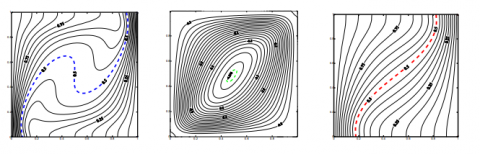(b)Horizontal magnetic field (Bx), Ha = 50

## 5c.png(c)Vertical magnetic field (By), Ha = 50

## 5d.png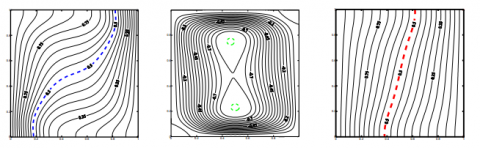(d)Horizontal magnetic field (Bx), Ha = 100

## 5e.png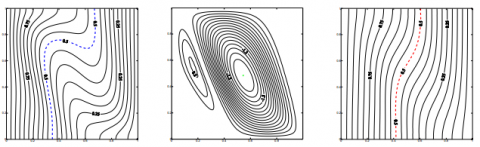(e)Vertical magnetic field (By), Ha = 100

Figure 5. Effect of magnetic field on the structure of the flow, isoconcentrations (left), current lines (center) and isotherms (right), for N = 0.75 oscillatory regime RaTCr= 1.01 × 105 at dimensionless time $\tau=5$

In fact, we go after the effect of magnetic field on the flow structure. For this, we present in Figure 5, the iso-concentrations, streamlines and isotherms for N = 0.75 in oscillatory regimes (RaTCr = 1.01 × 105) with a magnetic field applied along both horizontal and vertical directions corresponding to the numbers of Hartmann (Ha = 0, 50 and 100).

As results, we can see that, in oscillatory regimes, the magnetic field applied in the vertical direction stabilizes the flow better than the magnetic field applied in the horizontal direction. This is revealed in the dependent evolution of vertical dimensionless velocity and dimensionless concentration in the presence of a horizontal magnetic field Bx and vertical magnetic field By for Ha = 50, for point S2 and N = 0.75, RaTCr = 1.01 × 105 (Figure 6). We can note that the vertical magnetic field stabilizes the flow and becomes stable with low values of velocity obtained, this is mainly due to the interactions between the thermal and electromagnetic solutal forces, an intensity of the magnetic field (Ha = 50, vertical magnetic field), the flow slows down completely due to the strong dominance of the electromagnetic forces (Lorentz force).

## 6a.png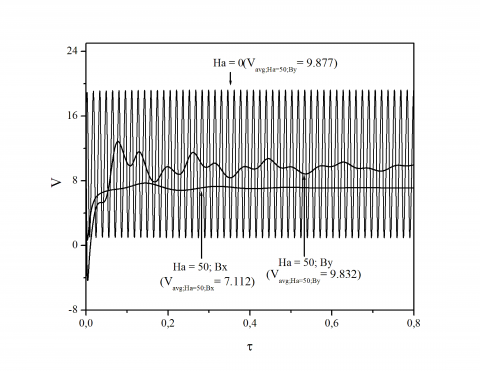(a)

## 6b.png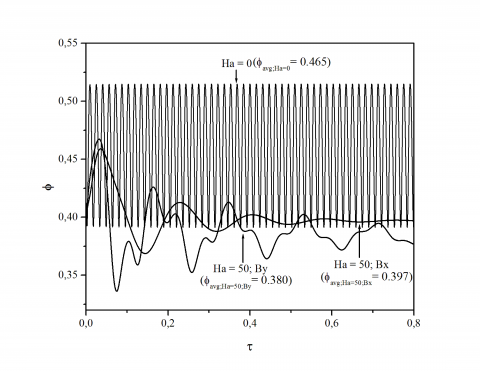(b)

Figure 6. Time dependent evolution of vertical dimensionless velocity (a) and dimensionless concentration (b) at monitoring points S2 (X = 0.39, Y = 0.39), in the presence of horizontal magnetic field and vertical magnetic field, for N = 0.75 ; RaTCr = 1.01 × 105, Ha = 0 and 50

In Figure 7, we present the variation of Nusselt number (Nu) and Sherwood number (Sh) for different Hartmann number (Ha) in oscillatory regimes (RaTCr = 1.01 × 105 and N = 0.75 ). The magnetic field was applied in the two horizontal directions (Bx) and vertical direction (By),   separately. We note that the values of Nusselt number and Sherwood number will decrease when the Hartmann number increases, for the two orientations of the magnetic field Bx and By. In addition, the vertical magnetic field shows a more decrease of these numbers in comparison with the magnetic field applied in the horizontal direction. This shows that the magnetic field is used to stabilize the double-diffusive convection flow, and the applied of magnetic field in the vertical direction provide the strong magnetic stabilization. These findings confirm those obtained by Chamkha and Al Naser .

## 7.pngFigure 7. Variation of the Nusselt number (Nu) and Sherwood number (Sh), for different values of the Hartmann number for N = 0.75 and RaTCr = 1.01 × 105 (oscillatory steady)

4.3 Effect of the magnetic field associated with the effect of the buoyancy ratio (N)

In this section, we are interested in examining the effect of the magnetic field on the double diffusive flow field for different values of buoyancy ratio forces N.

Three values of the buoyancy ratio were considered: an equilibrium value buoyancy force ( $N=1$ ), a value taken for the dominance of thermal forces ( $N \pi 1$ ), and finally a value taken for the case of the dominance of solutal force ( $N \phi 1$ ).

The magnetic field is applied in both horizontal and vertical directions separately, and the Hartmann number takes the following values: Ha = 0, 25, 50, 75 and 100 (Figure 8).

In each case, we determine the critical thermal Rayleigh number for each Hartmann number Ha, for two orientations of the magnetic field (Bx and By separately). We note that, the oscillatory regime is controlled in each case.

For Ha = 75 (magnetic field Bx) increases RaTCr 6.3701 × 105 ( N=0.75 ), and then increases RaTCr 106 (N=1) and reaches the critical thermal Rayleigh 3.001 × 106 (N=1.25). Then, the double diffusive flow stabilizes by the magnetic field.

Comparing the values of critical thermal Rayleigh number (RaTCr) and the dimensionless stream function shows that the value obtained by applying the vertical magnetic field (By) approves higher values than those obtained by the application of the horizontal magnetic field (Bx). For Ha = 100 (magnetic field Bx, N=0.75 ), RaTCr increases from 1.0021 × 106 to 1.01 × 106 by applying the vertical magnetic field (By) and N=0.75 . Then, for a magnetic field (Bx) (Ha = 100 and N = 1), RaTCr increases 3.5 × 106- 4.0 × 106 by applying the vertical magnetic field (By) and N = 1. Finally, for a magnetic field Bx (Ha = 100 and N=1.25 ), RaTCr increases from 5.5 × 106 to 6.0 × 106 by applying the vertical magnetic field (By) and N=1.25 .  So, we can conclude that the magnetic field provide the stabilization of double-diffusive oscillatory flow, and the vertical magnetic field stabilizes further and delay the transition of the regime of the flow of the double diffusion.

## 8.png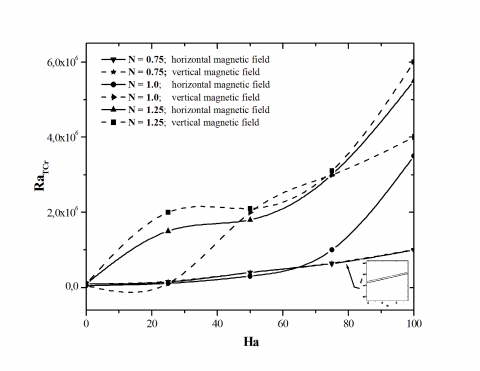Figure 8. Critical thermal Rayleigh number RaTcr versus Hartmann number, for N=0.75, 1 and 1.25 in the presence of horizontal magnetic field and vertical magnetic field By

5. Conclusion

A numerical study of double diffusive natural convection in binary mixture under the effect of external magnetic field for the steady and the oscillatory state was carried out. Opposing buoyancy forces due to horizontal thermal and concentration gradients were applied. Comparisons with previously published works were performed and found to be in good agreement. From the obtained results, the following conclusions are drawn:

•The dominance of thermal strengths ($N \pi 1.25$) leads to an increase of the intensity of the flow (stream function) better than those of the solutal strengths ($N \phi 1.25$).

•The application of the magnetic field showed a strong dependence of the critical thermal Rayleigh number and the Hartmann number Ha, for a given value of N.

•The vertical magnetic field provide the strong magnetohydrodynamic stabilisation and increases the critical thermal Rayleigh number RaTCr towards enough high values, what shows down the transition towards the instability of the flow of double diffusion which has incurable consequences on the production of semiconductors.

Nomenclature
 B intensity of magnetic field (Tesla) C solutal concentration (kg m-3) D molecular diffusivity (m2 s-1) FEMX component of Lorentz force in X direction FEMY component of Lorentz force in Y direction g gravitational acceleration (m s-2) k thermal conductivity (W m-1 K-1) L length of square cavity Nu Nusselt number p pressure (Pa) P dimensionless pressure Sc Schmidt number Sh Sherwood number T temperature (K) u, v velocity components (m s-1). U, V dimensionless velocity components x, y coordinates (m) X, Y dimensionless coordinates Greek symbols α thermal diffusivity (m2 s-1) βT thermal expansion coefficient (K-1) βC solutal expansion coefficient (kg-1 m3) ν kinematic viscosity (m2 s-1) γ orientation of the magnetic field, ° ρ density (kg. m-3) σ electric conductivity, Ω-1.m-1 τ dimensionless time θ dimensionless temperature Φ dimensionless concentration Subscripts Avg average value Cr critical value S solutal parameter T thermal parameter
References

1. D.T.J. Hurle, E. Jakeman and C.P. Johnson, “Convective temperature oscillation in molten gallium,” Journal of Fluid Mechanics, vol.64, pp.564-565, 1974. DOI: 10.1017/S0022112074002540.

2. B. Hof, A. Juel, and T. Mullin, “Magnetohydrodynamic damping of convection flows in molten gallium,” Journal of Fluid Mechanics, vol.482, pp.163-179, 2003. DOI: 10.1017/S0022112003004014.

3. K. Okada, and H. Ozoe, “Experimental heat transfer rates of natural convection of molten gallium suppressed under an external magnetic field in either the X, Y or Z direction,” ASME, Journal of Heat Transfer, vol.114, no.1, pp.107-114, 1992. DOI: 10.1115/1.2911234.

4. I. Di Pizza, and M. Ciofalo, “MHD free convection in a liquid-metal filled cubic enclosure. II. Internal heating,”International Journal of Heat and Mass Transfer, vol.45, pp.1493-1511, 2002. DOI: 10.1016/S0017-9310(01)00253-8.

5. S. Dost, Y.C. Liu, B. Lent, and R.F. Redden, “A numerical simulation study for the effect of applied magnetic field in growth of CdTe crystals by the travelling heater method,” International Journal of Applied Electromagnetics and Mechanics, vol.17, no.4, pp.271-288, 2003.DOI: 10.1016/S0022-0248(02)00830-8.

6. V. Galindo, G. Gerbeth, W. Ammon, E. Tomzig, and J. Virbulis, “Crystal growth melt flow control by means of magnetic field,” Energy Conversion and Management, vol.43, pp.309–316, 2002. DOI: 10.1016/S0196-8904(01)00100-5.

7. Y.C. Liu, Y. Okano, and S. Dost, “The effect of applied magnetic field on flow structure in liquid phase electroepitaxy- a three-dimensional simulation model,” Journal of Crystal Growth, vol.244, pp.12-26, 2002. DOI: 10.1016/S0022-0248(02)01603-2.

8. H. Sheibani, Y.C. Liu, S. Sakai, B. Lent, and S. Dost, “The effect of applied magnetic field on the growth mechanisms of liquid phase electroepitaxy,” International Journal of Engineering Sciences, vol.41, pp.401-415, 2003. DOI: 10.1016/S0020-7225(02)00219-7.

9. V. Kumar, S. Dost, and F. Durst, “Numerical modelling of crystal growth under strong magnetic fields: an application to the travelling heater method,” Applied Mathematic Modelling, vol.31, pp.589-605, 2007. DOI: 10.1016/j.apm.2005.11.022.

10. E. Yildiz, S. Dost, and M. Yildiz, “A numerical simulation study for the effect of magnetic fields in liquid phase diffusion growth of SiGe single crystals,” Journal of Crystal Growth, vol.291, pp.497-511, 2006. DOI: 10.1016/j.jcrysgro.2006.03.040.

11. N.Armour, and S.Dost, “Numerical and experimental study of forced mixing with static magnetic field on sige system,” FDMP: Fluid Dynamics and Materials Processing, vol.5 no.4, pp.331-344, 2009. DOI: 10.3970/fdmp.2009.005.331

12. A. YU Gelfgat and P. Z. Bar-Yoseph, “The effect of an external magnetic field on oscillatory instability of convective flows in a rectangular cavity,” Physics of Fluid, vol.13, no.8, pp.2269-2279, 2001. DOI: 10.1063/1.1383789.

13. R. Bessaih and S. Bouabdallah, “Numerical study of oscillatory natural convection during solidification of a liquid metal in a rectangular enclosure with and without magnetic field,” Numerical Heat Transfer, Part A., vol.54, pp.331-348, 2008. DOI: 10.1080/10407780802084660.

14. S. Bouabdallah and R. Bessaih, “Effect of magnetic field on 3D flow and heat transfer during solidification from a melt,” International Journal of Heat and Fluid Flow, vol.37, pp.154-166, 2012. DOI: 10.1016/j.ijheatfluidflow.2012.07.002.

15. B. Ghernaout, S. Bouabdallah, A. Benchatti and R. Bessaih, “Effect of the buoyancy ratio on oscillatory double-diffusive convection in binary mixture,” Numerical Heat Transfer, Part A, vol.66 no.8, pp.928-946, 2014. DOI: 10.1080/10407782.2014.892386.

16. S.V. Patankar, Numerical Heat Transfer and Fluid Flow, McGraw-Hill, New York, 1980.

17. C. Beghein, F. Haghighat and F. Allard, “Numerical study of double diffusive natural convection in a square cavity,” Int. J. Heat Mass Transfer, vol.35, no.4, pp.833-846, 1992. DOI: 10.1016/0017-9310(92)90251-M.

18. I. Sezai, and A.A. Mohamad, “Double diffusive convection in a cubic enclosure with opposing temperature and concentration gradients,” Physics of Fluids, vol.12 no.9, pp.2210-2223, 2000. DOI: 10.1063/1.1286422.

19. H. Han, and H.T. Kuehn, “Double diffusive natural convection in a vertical rectangular enclosure – II Numerical study,” Int. J. Heat and Mass Transfer, vol.34, no.2, pp.461-471, 1991a. DOI: 10.1016/0017-9310(91)90265-G.

20. H. Han, and H.T. Kuehn, “Double diffusive natural convection in a vertical rectangular enclosure- I. Experimental study,” Int. J. Heat and Mass Transfer, vol.34, no.2, pp.449-459, 1991b. DOI: 10.1016/0017-9310(91)90264-F.

21. H. Zhou, and A. Zebib, “Oscillatory double-diffusive convection in crystal growth,” Journal of Crystal Growth, vol.135, pp.587-593, 1994. DOI: 10.1016/0022-0248(94)90151-1.

22. A.J. Chamkha, and H. Al-Naser, “Hydromagnetic double-diffusive convection in a rectangular enclosure with opposing temperature and concentration gradients,” International Journal of Heat and Mass Transfer, vol.45, pp.2465–2483, 2002. DOI: 10.1016/S0017-9310(01)00344-1.